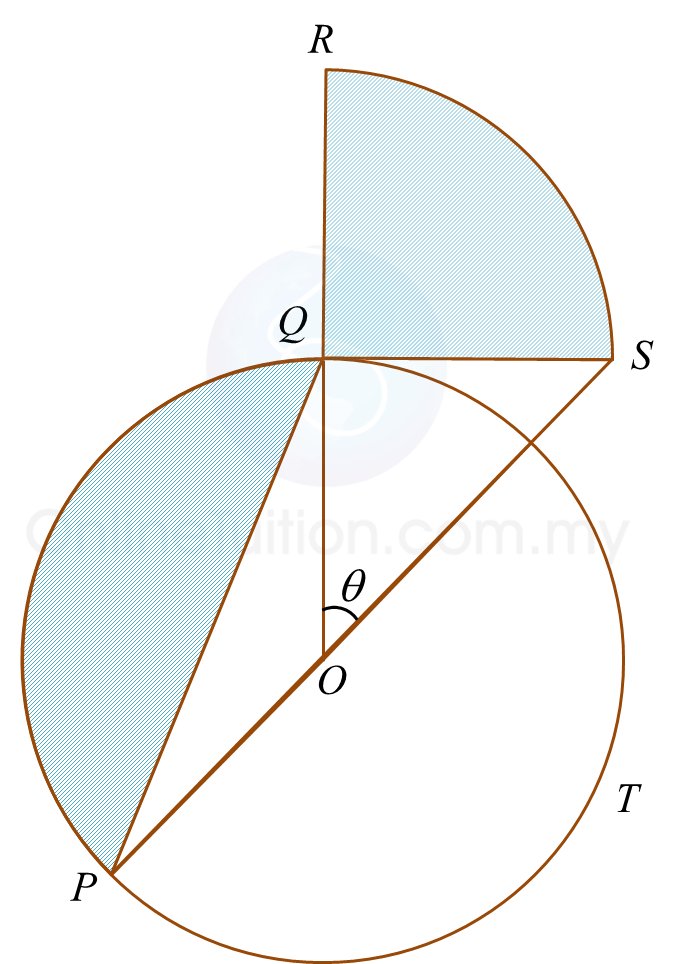# 1.5.4 Circular Measure, Long Questions (Question 7 & 8)

Question 7:
Diagram below shows a semicircle PTS, centre O and radius 8 cm. PTR is a sector of a circle with centre P and Q is the midpoint of OS.[Use π = 3.142]
Calculate
(b) the length , in cm , of the arc TR ,
(c) the area, in cm2 ,of the shaded region.

Solution:
(a)

(b)(c)

Question 8:
Diagram below shows a circle PQT with centre O and radius 7 cm.QS
is a tangent to the circle at point Q and QSR is a quadrant of a circle with centre Q. Q is the midpoint of OR and QP is a chord. OQR and SOP are straight lines.
[Use π = 3.142]
Calculate